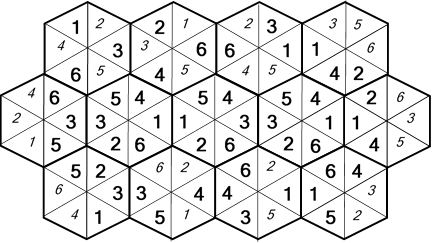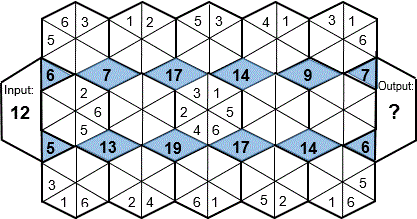Select PagePrintable version

HEXAGON-numeric (basic)

Fit the numbers 1 – 6 in each hexagon. Where the sides of different hexagons meet, the adjoining segments will have the same number value. No number can be repeated in a hexagon.### SolutionHEXAGON-numeric (intermediate)

Fit the numbers 1 – 6 in each hexagon. Where the sides of different hexagons meet, the adjoining segments will have the same number value. No number can be repeated in a hexagon. The numbers in the shaded areas are the sum of the numbers in the 2 or 4 segments that they adjoin. The numbers in the ‘Input’ and ‘Output’ boxes are the sum of the numbers in the 3 triangular segments that they side with. Solve the puzzle and find the ‘Output’ number (?).### SolutionFit the numbers 1 – 6 in each hexagon. Where the sides of different hexagons meet, the adjoining segments will have the same number value. No number can be repeated in a hexagon. The numbers in the shaded areas are the sum of the numbers in the 2 or 4 segments that they adjoin. The numbers in the ‘Input’ and ‘Output’ boxes are the sum of the numbers in the 3 triangular segments that they side with. The numbers in the ‘Sum’ boxes are the sum of the numbers in the 4 segments that they side with. Solve the puzzle and find the Output’ number (?).### Solution## Feedback

There are more than one way of doing these puzzles and may well be more than one answer.  Please let me and others know what alternatives you find by commenting below.  We also welcome general comments on the subject and any feedback you’d like to give.   If you have a question that need a response from me or you would like to contact me privately, please use the contact form.

## Get more puzzles!

If you’ve enjoyed doing the puzzles, consider ordering the book; 150+ of the best puzzles in a handy pocket sized format. Click here for full details.